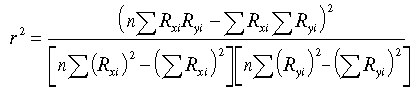# R-Squared

Financial analysis Print Email

Definition of R-Squared

R-squared is a statistical measure that provides with data in percentage of a fund from the standard index or by definition the value of fraction of variance. The value of R-squared can vary from 0 to 100. If the R-squared of a security is 100, it denotes that all the movements of security are completely ascertained by the standard movement of market index.

Similarly, a high R-square in the range between 85 and 100 would denote that fund’s performance are along the standard lines of index and the funds low r-squared index which is in the range of 70 and less indicates that the funds are not performing at par with the standard index.

If the r-squared value is high, it indicates how useful its beta figure is. If a fund with r-squared value is 100 or in a higher range but has a beta which is below 1 then it is offering a high risk-adjusted return, and low r-squared value would not have any standing in the market, so it is best to ignore the beta of the low r-squared fund.

In another definition, R-squared is the ratio of changeability of the modeled values to the changeability of the original data ideals. By yet another definition which is relating to the modeled values which are obtained by ordinary smallest amount squares degeneration gives r-squared value as the square of the correlation between the new and modeled data values.

How to Calculate R-Squared

R-squared helps in ascertaining the degree of correlation of a company or a single stock and the market or other stocks. This analysis would allow investors to predict the future market trends and how the stock would grow in the coming future.

The formula which is used to measure R-squared value isWhere,

r 2= R-squared

n = number of observations

Rxi = Market excess return
(market proxy return minus risk-free proxy return)

Ryi= Portfolio's excess return
(portfolio return minus risk free proxy return)

This formula helps in ascertaining the extent to which the fluctuations of a group’s returns are correlated with the returns of the market in general. An r-squared value of 1.0 (100%) indicates a perfect fit of the market. And 0 is the worst that you can have as an R-squared value. R-squared value does not indicate if the appropriate set of variables that are chosen and the regression was used.